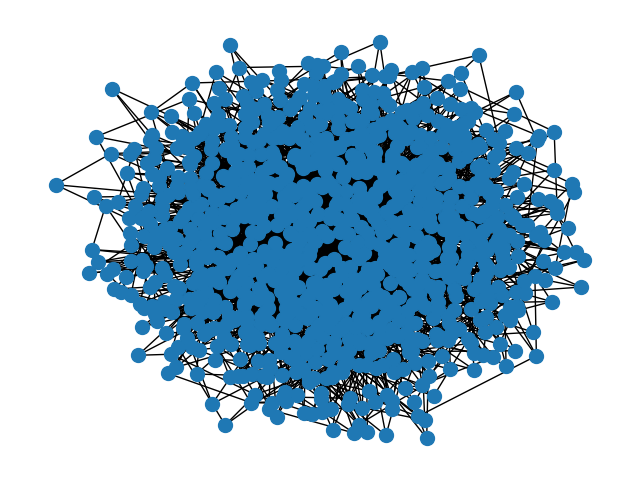# 平行中间值¶Computing betweenness centrality for:
Name:
Type: Graph
Number of nodes: 1000
Number of edges: 2991
Average degree:   5.9820
Parallel version
Time: 3.4363
Betweenness centrality for node 0: 0.00096
Non-Parallel version
Time: 3.8495 seconds
Betweenness centrality for node 0: 0.00096

Computing betweenness centrality for:
Name:
Type: Graph
Number of nodes: 1000
Number of edges: 4887
Average degree:   9.7740
Parallel version
Time: 4.1927
Betweenness centrality for node 0: 0.00382
Non-Parallel version
Time: 5.4284 seconds
Betweenness centrality for node 0: 0.00382

Computing betweenness centrality for:
Name:
Type: Graph
Number of nodes: 1000
Number of edges: 2000
Average degree:   4.0000
Parallel version
Time: 3.4163
Betweenness centrality for node 0: 0.00586
Non-Parallel version
Time: 3.5349 seconds
Betweenness centrality for node 0: 0.00586


from multiprocessing import Pool
import time
import itertools

import matplotlib.pyplot as plt
import networkx as nx

def chunks(l, n):
"""Divide a list of nodes l in n chunks"""
l_c = iter(l)
while 1:
x = tuple(itertools.islice(l_c, n))
if not x:
return
yield x

def betweenness_centrality_parallel(G, processes=None):
"""Parallel betweenness centrality  function"""
p = Pool(processes=processes)
node_divisor = len(p._pool) * 4
node_chunks = list(chunks(G.nodes(), int(G.order() / node_divisor)))
num_chunks = len(node_chunks)
bt_sc = p.starmap(
nx.betweenness_centrality_source,
zip([G] * num_chunks, [True] * num_chunks, [None] * num_chunks, node_chunks),
)

# Reduce the partial solutions
bt_c = bt_sc
for bt in bt_sc[1:]:
for n in bt:
bt_c[n] += bt[n]
return bt_c

if __name__ == "__main__":
G_ba = nx.barabasi_albert_graph(1000, 3)
G_er = nx.gnp_random_graph(1000, 0.01)
G_ws = nx.connected_watts_strogatz_graph(1000, 4, 0.1)
for G in [G_ba, G_er, G_ws]:
print("")
print("Computing betweenness centrality for:")
print(nx.info(G))
print("\tParallel version")
start = time.time()
bt = betweenness_centrality_parallel(G)
print("\t\tTime: %.4F" % (time.time() - start))
print("\t\tBetweenness centrality for node 0: %.5f" % (bt))
print("\tNon-Parallel version")
start = time.time()
bt = nx.betweenness_centrality(G)
print("\t\tTime: %.4F seconds" % (time.time() - start))
print("\t\tBetweenness centrality for node 0: %.5f" % (bt))
print("")

nx.draw(G_ba)
plt.show()


Total running time of the script: ( 0 minutes 31.318 seconds)

Gallery generated by Sphinx-Gallery